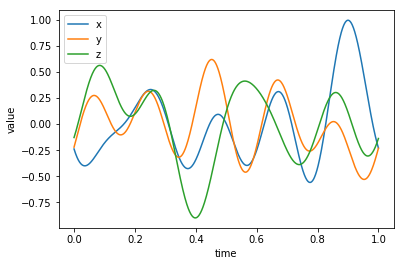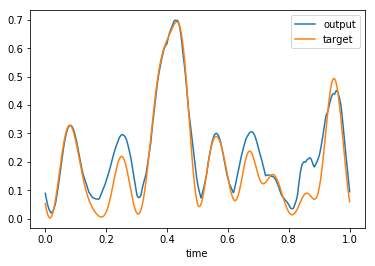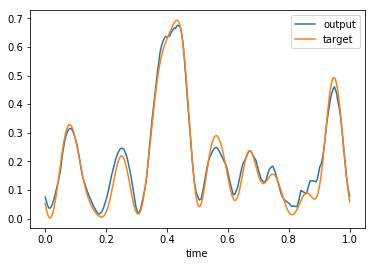# Optimizing the parameters of a Nengo model¶

Nengo uses the Neural Engineering Framework to optimize the parameters of a model. NengoDL adds a new set of optimization tools (deep learning training methods) to that toolkit, which can be used instead of or in addition to the NEF optimization.

Which techniques work best will depend on the particular model being developed. However, as a general rule of thumb, the gradient-descent based deep learning optimizations will tend to provide more accurate network output, but take longer to optimize and require the network to be differentiable.

Here we’ll go through an example showing how a Nengo model can be optimized using these training tools. We’ll build a network to compute the arbitrarily chosen function $$f(x, y, z) = (x+1)*y^2 + \sin(z)^2$$.

In :

%matplotlib inline

import nengo
import nengo_dl
import matplotlib.pyplot as plt
import numpy as np
import tensorflow as tf


We’ll begin by setting some default parameters for our network. These parameters have been chosen to make the training easier/faster for this example.

In :

net = nengo.Network()
net.config[nengo.Ensemble].neuron_type = nengo.RectifiedLinear()
net.config[nengo.Ensemble].max_rates = nengo.dists.Uniform(0, 1)
net.config[nengo.Connection].synapse = None


Next we’ll define the inputs for our network. These could be whatever we want, but for this example we’ll use band-limited white noise.

In :

with net:
x, y, z = [nengo.Node(output=nengo.processes.WhiteSignal(1, 5, rms=0.3, seed=i))
for i in range(3)]

plt.figure()
plt.plot(np.linspace(0, 1, 1000), x.output.run(1.0), label="x")
plt.plot(np.linspace(0, 1, 1000), y.output.run(1.0), label="y")
plt.plot(np.linspace(0, 1, 1000), z.output.run(1.0), label="z")
plt.xlabel("time")
plt.ylabel("value")
plt.legend();Now we’re ready to define the structure of our network. We’ll create three ensembles; one will compute $$(x+1)*y^2$$, another will compute $$\sin(z)$$, and the third will square the output of the previous population to compute $$\sin(z)^2$$. Again, there are various different network structures we could have chosen to compute this function, this is just one of them.

In :

with net:
# neural ensembles
ens0 = nengo.Ensemble(100, 2)
ens1 = nengo.Ensemble(50, 1)
ens2 = nengo.Ensemble(50, 1)

# connect the input signals to ensemble inputs
nengo.Connection(x, ens0)
nengo.Connection(y, ens0)
nengo.Connection(z, ens1)

# output node
f = nengo.Node(size_in=1)

# create a connection to compute (x+1)*y^2
nengo.Connection(ens0, f, function=lambda x: (x + 1) * x ** 2)

# create a connection to compute sin(z)
nengo.Connection(ens1, ens2, function=np.sin)

# create a connection to compute sin(z)^2
nengo.Connection(ens2, f, function=np.square)

# collect data on the inputs/outputs
x_p = nengo.Probe(x)
y_p = nengo.Probe(y)
z_p = nengo.Probe(z)
f_p = nengo.Probe(f)


When we build this network the NEF optimization will be used to compute the weights on each connection, based on the functions we specified. If we run the network we can see that the network does a pretty good job of approximating the target function.

In :

def target_func(x, y, z):
return (x + 1) * y ** 2 + np.sin(z) ** 2

with nengo_dl.Simulator(net) as sim:
sim.run(1.0)

plt.figure()
plt.plot(sim.trange(), sim.data[f_p], label="output")
plt.plot(sim.trange(), target_func(sim.data[x_p], sim.data[y_p],
sim.data[z_p]), label="target")
plt.legend()
plt.xlabel("time")

Building network
Build finished in 0:00:00
Optimization finished in 0:00:00
Construction finished in 0:00:00
Simulation finished in 0:00:01To apply further optimization, using deep learning methods, we first need to specify a training data set. This defines the input values (for $$x$$, $$y$$, and $$z$$), and the output value we expect for each set of input values. Each input should have shape (number of training examples, number of simulation timesteps, input dimensionality); in this case we’ll create a dataset with 1024 training examples, each of our inputs are 1D, and we only need to train for one timestep at a time (since our network doesn’t have any temporal dynamics). The inputs are specified as a dictionary mapping Nodes to input values, and the targets as a dictionary mapping Probes to target values. We’ll use random uniform numbers from -1 to 1 as our input data, so our inputs and targets will look like

In :

inputs = {x: np.random.uniform(-1, 1, size=(1024, 1, 1)),
y: np.random.uniform(-1, 1, size=(1024, 1, 1)),
z: np.random.uniform(-1, 1, size=(1024, 1, 1))}

targets = {f_p: target_func(inputs[x], inputs[y], inputs[z])}


We can use the sim.loss function to check the initial error for our network on this data. We’ll use mean-squared-error (MSE) as our error measure (see the documentation for more detail on specifying different error functions). Note that we’ll also re-build the model with minibatch_size=32 (so that we can process the 1024 inputs in chunks of 32 rather than one at a time).

In :

sim.close()
sim = nengo_dl.Simulator(net, minibatch_size=32, device="/cpu:0")

print("pre-training mse:", sim.loss(inputs, targets, "mse"))

Building network
Build finished in 0:00:00
Optimization finished in 0:00:00
Construction finished in 0:00:00
pre-training mse: 0.009830936229263898


Next we need to define the optimization method we’ll use to train the model. Any TensorFlow optimizer can be used; here we’ll use gradient descent with Nesterov momentum.

In :

opt = tf.train.MomentumOptimizer(learning_rate=0.002, momentum=0.9, use_nesterov=True)


We’re now ready to train the model. The last thing we need to specify is the number of epochs we want to train for, where each epoch is one complete pass through the training data.

In :

sim.train(inputs, targets, opt, n_epochs=100)

Training finished in 0:00:07 (loss: 0.0007)


If we check the error after the training, we can see that it has improved significantly.

In :

print("post-training mse:", sim.loss(inputs, targets, "mse"))

post-training mse: 0.0008054008176259231


And we can confirm this by running the model again and plotting the results:

In :

sim.run(1.0)

plt.figure()
plt.plot(sim.trange(), sim.data[f_p], label="output")
plt.plot(sim.trange(), target_func(sim.data[x_p], sim.data[y_p],
sim.data[z_p]), label="target")
plt.legend()
plt.xlabel("time")

Simulation finished in 0:00:00

Out:

Text(0.5,0,'time')In :

sim.close()